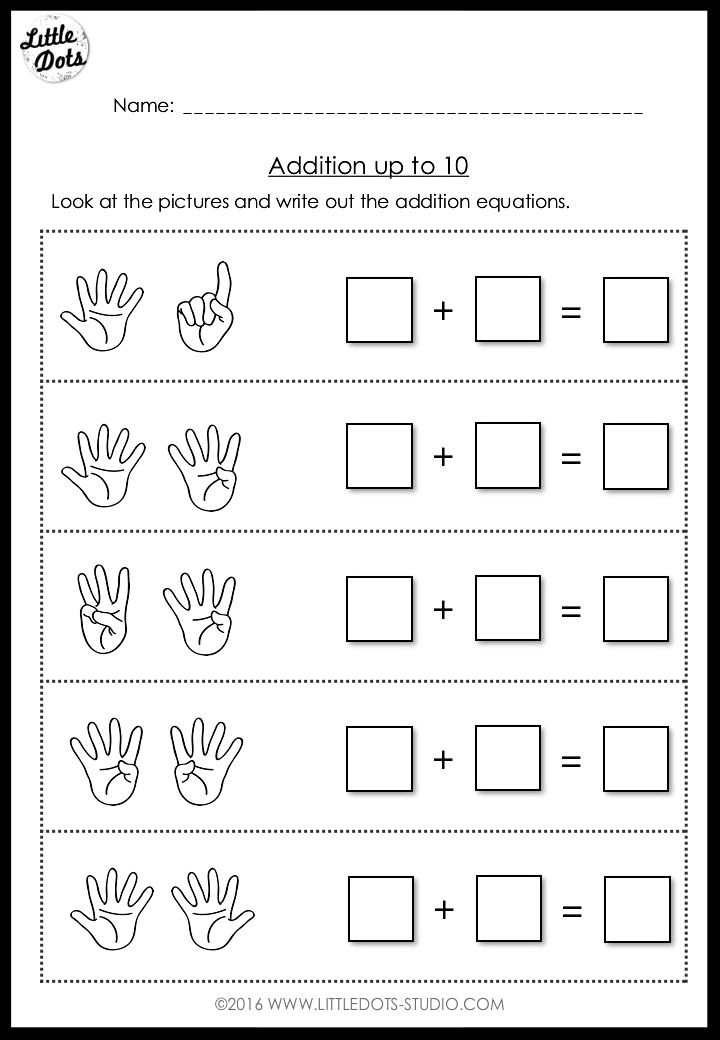top of pageSearch
• Ronia

# Kindergarten Addition within 10 Worksheets and Activities

Updated: Jan 31, 2022

This addition bundle includes 20 worksheets and activities on addition within 10 suitable for kindergarten to grade 1 level. These activities help children to:

• Understand the concept of adding one/ two more

• Practice to solve addition equations within 10 using pictures (e.g. objects, domino tiles, dice, tally marks, fingers and ten frame)

• Learn to use number line to help solve addition problems

• Practice writing out addition equations from pictures given

• Create their own addition equations

• Explore various way to make up 10 using ten frames

• 6 worksheets to help children understand the term 'and', perfect for introduction to addition. Check out some of the sample worksheets below.• 2 worksheets to practice solving addition equations with dots• 4 worksheets to practice writing out addition equations from pictures and ten frames• 2 worksheets to create own addition equations​• 2 worksheets to practice solving addition equations using number line